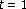# Positive Solutions for Nonlinearth-Order Singular Nonlocal Boundary Value Problems

## Abstract

We study the existence and multiplicity of positive solutions for a class ofth-order singular nonlocal boundary value problems,,where. The singularity may appear atand/or. The Krasnosel'skii-Guo theorem on cone expansion and compression is used in this study. The main results improve and generalize the existing results.

## References

1. Gupta CP: Solvability of a three-point nonlinear boundary value problem for a second order ordinary differential equation. Journal of Mathematical Analysis and Applications 1992,168(2):540–551. 10.1016/0022-247X(92)90179-H

2. Il'in VA, Moiseev EI: A nonlocal boundary value problem of the first kind for the Sturm-Liouville operator in differential and difference interpretations. Differential Equations 1987,23(7):803–810.

3. Eloe PW, Henderson J: Positive solutions for higher order ordinary differential equations. Electronic Journal of Differential Equations 1995,1995(3):8 pages.

4. Ma RY: Positive solutions for a nonlinear three-point boundary-value problem. Electronic Journal of Differential Equations 1999,1999(34):8 pages.

5. O'Regan D: Singular second order boundary value problems. Nonlinear Analysis 1990,15(12):1097–1109. 10.1016/0362-546X(90)90046-J

6. Hao Z-C, Mao AM: A necessary and sufficient condition for the existence of positive solutions to a class of singular second-order boundary value problems. Journal of Systems Science and Mathematical Sciences 2001,21(1):93–100.

7. Agarwal RP, O'Regan D: Positive solutions to superlinear singular boundary value problems. Journal of Computational and Applied Mathematics 1998,88(1):129–147. 10.1016/S0377-0427(97)00205-7

8. Ma RY: Positive solutions of singular second-order boundary value problems. Acta Mathematica Sinica 1998,41(6):1225–1230.

9. Hao Z-C, Liang J, Xiao T-J: Positive solutions of operator equations on half-line. Journal of Mathematical Analysis and Applications 2006,314(2):423–435. 10.1016/j.jmaa.2005.04.004

10. Kiguradze IT, Lomtatidze AG: On certain boundary value problems for second-order linear ordinary differential equations with singularities. Journal of Mathematical Analysis and Applications 1984,101(2):325–347. 10.1016/0022-247X(84)90107-0

11. Lomtatidze AG: A boundary value problem for second-order nonlinear ordinary differential equations with singularities. Differential Equations 1986,22(3):416–426.

12. Lomtatidze AG: Positive solutions of boundary value problems for second-order ordinary differential equations with singularities. Differential Equations 1987,23(10):1146–1152.

13. Eloe PW, Ahmad B: Positive solutions of a nonlinearth order boundary value problem with nonlocal conditions. Applied Mathematics Letters 2005,18(5):521–527. 10.1016/j.aml.2004.05.009

14. Guo DJ, Lakshmikantham V: Nonlinear Problems in Abstract Cones, Notes and Reports in Mathematics in Science and Engineering. Volume 5. Academic Press, Boston, Mass, USA; 1988.

## Author information

Authors

### Corresponding author

Correspondence to Xin'an Hao.

## Rights and permissions

Reprints and Permissions

Hao, X., Liu, L. & Wu, Y. Positive Solutions for Nonlinearth-Order Singular Nonlocal Boundary Value Problems. Bound Value Probl 2007, 074517 (2007). https://doi.org/10.1155/2007/74517

• Revised:

• Accepted:

• Published:

• DOI: https://doi.org/10.1155/2007/74517

### Keywords

• Differential Equation
• Partial Differential Equation
• Ordinary Differential Equation
• Functional Equation
• Nonlocal Boundary